# Osculating paraboloid

(diff) ← Older revision | Latest revision (diff) | Newer revision → (diff)
Jump to: navigation, search

of a surface at a pointThe paraboloid that reproduces the shape of the surface near this point up to variables of the second order of smallness with respect to the distance from. Letbe a paraboloid (see Fig.) with vertexand tangent to the surface at this point, and letandbe the distances of an arbitrary pointon the paraboloid to the surface and to, respectively.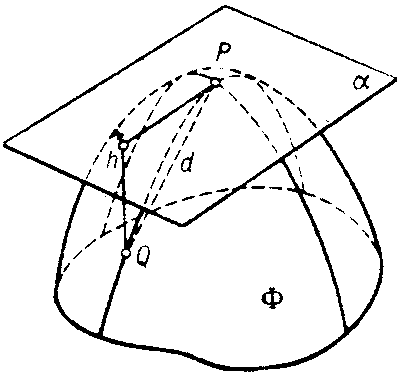Figure: o070550a

Thenis said to osculate ifas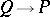. This does not exclude the degeneration of the paraboloid into a parabolic cylinder or plane. At every point of a regular surface there is a unique osculating paraboloid. Osculating paraboloids can be used to classify the points on a surface (see Elliptic point; Hyperbolic point; Parabolic point; Flat point).

#### Comments

The osculating paraboloid atto the surfacehas contact of order three withat, i.e. the derivatives up to and including order 2 of the difference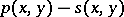of the functions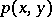anddescribing the paraboloid and the surface are all zero at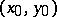, where.

How to Cite This Entry:
Osculating paraboloid. Encyclopedia of Mathematics. URL: http://encyclopediaofmath.org/index.php?title=Osculating_paraboloid&oldid=12753
This article was adapted from an original article by D.D. Sokolov (originator), which appeared in Encyclopedia of Mathematics - ISBN 1402006098. See original article